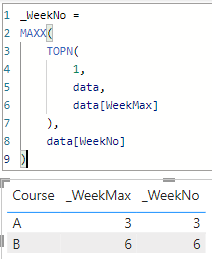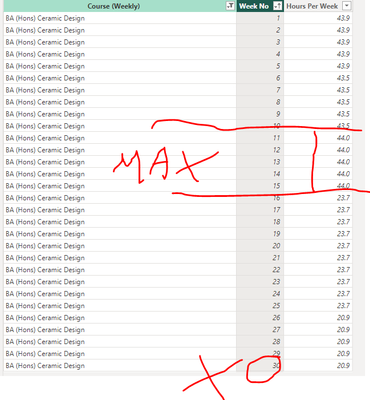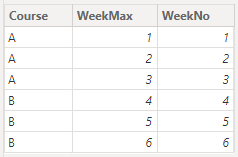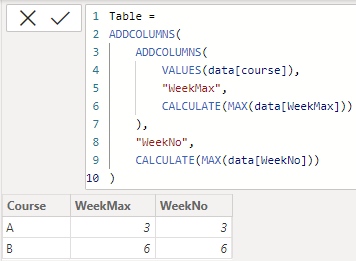cancel
Showing results for
Did you mean:Helper I

## Return Week number of Max Events

Hi, need help with a DAX Query I want to retune which week(s) the max hours occurs not all weeks for each course?

Weekly Course Hours Max = SUMMARIZECOLUMNS('Weekly Course Hours'[Course (Weekly)],'Weekly Course Hours'[Week No],"Max Week" , MAX('Weekly Course Hours'[Hours Per Week]))1 ACCEPTED SOLUTIONCommunity Champion

or you plot the course column with two measures like:

``_WeekMax = MAX(data[WeekMax])``
``````_WeekNo =
MAXX(
TOPN(
1,
data,
data[WeekMax]
),
data[WeekNo]
)``````

it worked like:4 REPLIES 4Helper I

Thanks, it is common thet the max week occurs more then once, is there a war to expemf to reuurm them all

i.e. Course A may have a max hours in week 3,7 and week 10.Helper I

Hi, Thanks, nearly right

It is returning the hours for the max week, but it is returning the last (Max) value for Week No. and not the weeks this values occur on i,e, the first course has 30 weeks of data, so it is returning 30 and not weeks 11, 12, 14 14 & 15Community Champion

hi @Garbo62

not sure if i fully get you, supposing you have a data table like:try to create a calculated table like:

``````Table =
VALUES(data[course]),
"WeekMax",
CALCULATE(MAX(data[WeekMax]))
),
"WeekNo",
CALCULATE(MAX(data[WeekNo]))
)``````

it worked like:Community Champion

or you plot the course column with two measures like:

``_WeekMax = MAX(data[WeekMax])``
``````_WeekNo =
MAXX(
TOPN(
1,
data,
data[WeekMax]
),
data[WeekNo]
)``````

it worked like: